# Associative rings and algebras

(diff) ← Older revision | Latest revision (diff) | Newer revision → (diff)

Rings and algebras with an associative multiplication, i.e. sets with two binary operations, addition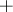and multiplication, that are Abelian groups with respect to addition and semi-groups with respect to multiplication, and in which the multiplication is distributive (from the left and from the right) with respect to the addition. Moreover, an associative algebra should be a vector space over a fixed field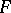, and the multiplication in it is compatible with multiplication by field elements in the sense of the condition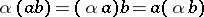for alland all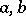from the algebra.

The first examples of associative rings and associative algebras were number rings and fields (the field of complex numbers and its subrings), polynomial algebras, matrix algebras over fields, and function fields. The theory of associative rings and algebras became an independent part of algebra at the beginning of the 20th century. This theory has many contact points with numerous fields of mathematics, especially so with algebraic geometry and algebraic number theory (commutative rings), functional analysis (commutative normed rings, operator rings and function rings), and topology (rings of continuous functions on topological spaces). The theory of fields, the theory of commutative rings (cf. Field; Commutative ring, see also Commutative algebra) and the theory of representations of associative algebras have become independent branches of the theory of associative rings and algebras. The theory of topological rings and skew-fields forms part of topological algebra.

The classical part of the theory of associative rings and algebras is formed by the theory of finite-dimensional associative algebras . The principal results of this theory are: A finite-dimensional simple associative algebra (i.e. one without proper ideals) over a fieldis a complete matrix algebra over some skew-field that is finite-dimensional over(Wedderburn's theorem); a finite-dimensional associative algebra over a field of characteristic zero (and, even more generally — a separable finite-dimensional associative algebra) is the direct sum (as linear spaces) of its radical I (i.e. its maximal nilpotent ideal) and some semi-simple (i.e. with a radical zero) subalgebra, any two complementary semi-simple subalgebrasand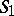being conjugate (cf. Wedderburn–Mal'tsev theorem).

One of the most important classes of associative algebras are skew-fields (cf. Skew-field, i.e. associative rings in which the equations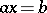and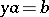are solvable for all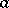,in the ring,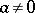). Skew-fields that are algebras over a certain field are called division algebras (cf. Division algebra). The theory of finite-dimensional division algebras forms a classical part of the theory of fields. All finite-dimensional associative division algebras over the field of real numbers have been described: these are the field of real numbers, the field of complex numbers and the skew-field of quaternions (cf. Frobenius theorem). All finite skew-fields are commutative (Wedderburn's theorem on skew-fields). A Galois theory of skew-fields has been constructed .

The key concepts in the structural theory of associative rings are the concepts of the Jacobson radical, semi-simplicity and primitivity. An associative ring is called semi-simple (in the sense of Jacobson) if its Jacobson radical is zero. A ring is called primitive (from the right) if it has an irreducible exact right module. All semi-simple associative rings are a subdirect sum of primitive rings. Any primitive associative ringis a dense ring of linear transformations of some vector spaceover a skew-field (Jacobson's density theorem); here the meaning of density is that for all linearly independent elements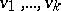fromand all elementsfromthere exists a transformation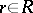such that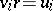for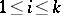. The general theory of radicals occupies an important place in the structural theory of rings (cf. Radical of rings and algebras).

The theory of Artinian rings (from the right, cf. Artinian ring), i.e. rings with the descending chain condition (minimum condition) for right ideals, forms a classical part of the theory of associative rings. The main result of this theory is that an associative ring is a semi-simple Artinian ring if and only if it is a direct sum of a finite number of complete matrix rings over skew-fields (the Wedderburn–Artin theorem).

The concept of the (classical) ring of fractions is important in the structural theory of associative rings. A ring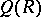is called the (right) ring of fractions of a subring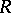of it if inall the regular elements (i.e. not divisors of zero) ofare invertible and if any element ofhas the form, where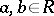. An associative ring has a ring of fractions if and only if for all elements, where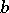is regular, there exist elementssuch that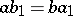and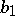is regular (Ore's theorem). A ringhas a semi-simple Artinian ring of fractions if and only if it is semi-primary (i.e.for all non-zero ideals), satisfies the minimum condition for right annihilating ideals of the form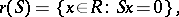whereis a subset of, and contains no infinite direct sums of right ideals (Goldie's theorem). In addition to the classical rings of fractions, rings of fractions having other meanings are also studied — mainly maximal or complete rings of fractions .

Special stress is laid on the study of free associative algebras (cf. Free associative algebra). Letbe a field and letbe a set. The free associative algebra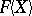overwith a unit element and with baseis the algebra of non-commutative polynomials with free terms in the set of variablesand with coefficients from. The algebrais characterized by the fact that it is is generated byas an algebra with a unit element and any mapping ofinto an associative algebrawith a unit element can be extended (even uniquely) to a homomorphism frominto. A free associative algebra is a ring with free ideals, i.e. the right (left) ideals of the ringare free right (left)-modules, and all bases of the free finitely-generated-modules contain the same number of elements (Cohn's theorem). Other examples of rings with free ideals are group algebras of free groups, and free products of associative division algebras. The free associative algebrais also a unique factorization domain: Any non-invertible elementhas a representation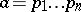, where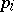are irreducible elements, and this representation is unique except for the order of the sequence and similarities (two elementandof a ringare called similar ifandare isomorphic as right-modules). The centralizer of each non-scalar element of the algebrais isomorphic to the algebra of polynomialsin one variable(Bergman's theorem).

Group algebras and PI-algebras are important classes of associative algebras (cf. Group algebra; PI-algebra). The theory of varieties of rings is in the process of being developed.

The importance of ring theory in mathematics has increased with the development of homological algebra. Many known classes of rings may be characterized in terms of properties of the category of modules (cf. Modules, category of) over these rings. For instance, a ringis a semi-simple Artinian ring if and only if all right (left) modules overare projective (injective). A ringis regular (in the sense of von Neumann) if and only if all right (left) modules overare flat. See also Regular ring (in the sense of von Neumann); Homological classification of rings; Quasi-Frobenius ring.